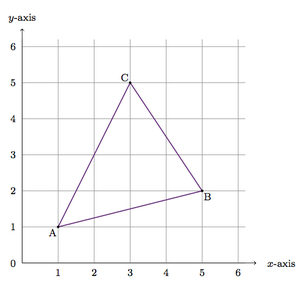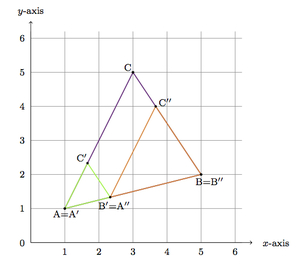# Scaling a Triangle in the Coordinate Plane

Alignments to Content Standards: G-GPE.B.6

Below is a picture of $\triangle ABC$ with vertices lying on grid points:1. Draw the image of $\triangle ABC$ when it is scaled with a scale factor of $\frac{1}{3}$ about the vertex $A$: label this triangle $A^\prime B^\prime C^\prime$ and find the coordinates of the points $A^\prime$, $B^\prime$, and $C^\prime$.
2. Draw the image of $\triangle ABC$ when it is scaled with a scale factor of $\frac{2}{3}$ about the vertex $B$: label this triangle $A^{\prime \prime}B^{\prime \prime}C^{\prime \prime}$ and find the coordinates of the points $A^{\prime \prime}$, $B^{\prime \prime}$, and $C^{\prime \prime}$.
3. How does $A^{\prime \prime}$ compare $B^{\prime}$? Why?

## IM Commentary

The goal of this task is to apply dilations to a triangle in the coordinate plane. Students will need to find the coordinates of a point which cuts a given segment into one third (part a) and into two thirds (part b). Because the centers for the two dilations are vertices of $\triangle ABC$ and the sum of the dilation factors (one third and two thirds) is one, the two dilated triangles share a vertex. A more challenging question, in place of (b) and (c), would be to ask students which dilations they can apply about $B$ so that the image will share a vertex with the triangle constructed in part (a): there are two possibilities, namely $\frac{2}{3}$ and 1.

## Solution

1. Since $A$ is the center of the dilation, it does not move and we will have $A^\prime = A$. For vertex $B$, we know that $B^\prime$ will lie on $\overleftrightarrow{AB}$ because dilations preserve lines through the center of dilation. Since the scale factor is $\frac{1}{3}$ we know that $|AB^\prime| = \frac{1}{3}|AB|$. From $A$ to $B$ is 4 units to the right and one unit up. Therefore, one third of the way from $A$ to $B$ (along $\overline{AB}$) will be the point $B^\prime = \left(1+ \frac{4}{3}, 1 + \frac{1}{3}\right)$. Applying this technique to $C$ which is 2 units to the right and 4 units up from $A$, we find $$C^\prime = \left(1\frac{2}{3}, 2\frac{1}{3} \right).$$

The scaled triangle $A^\prime B^\prime C^\prime$ is pictured below:2. Since $B$ is the center of the dilation, it does not move and we will have $B^{\prime \prime} = B$. For vertex $A$, we know that $A^{\prime \prime}$ will lie on $\overleftrightarrow{AB}$ because dilations preserve lines through the center of dilation. Since the scale factor is $\frac{2}{3}$ we know that $|BA^{\prime \prime}| = \frac{2}{3}|BA|$. From $B$ to $A$ is 4 units to the left and one unit down. Therefore, two thirds of the way from $B$ to $A$ (along $\overline{AB}$) will be the point $A^{\prime \prime} = \left(5 - \frac{8}{3}, 2 - \frac{2}{3}\right)$. Applying this technique to $C$ which is 2 units to the left and 3 units up from $B$, we find $$C^{\prime \prime} = \left(3\frac{2}{3}, 4 \right).$$

The scaled triangle $A^{\prime \prime} B^{\prime \prime} C^{\prime \prime}$ is pictured below:3. The calculations above show that $B^\prime = A^{\prime \prime} = \left( 2 \frac{1}{3}, 1 \frac{1}{3} \right)$. To see why these vertices are the same, note that the sum of the dilation factors $\frac{1}{3}$ and $\frac{2}{3}$ is 1. The dilation about $A$ maps $\overline{AB}$ to a the first third of $\overline{AB}$ while the dilation about $B$ maps $\overline{AB}$ to the second two thirds of this segment. The two scaled triangles with a shared vertex are pictured below: